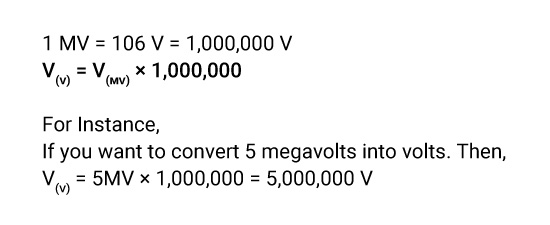## About Our MV to V Converter

"Zeroes must be placed in the right place in the right number to give a correct value in metric conversion."

When converting from imperial to metric, several zeroes often sets one to confusion. Measurement results will undoubtedly get wrong if there is one less or more zero. Therefore, we proudly present a handy converter that allows you to convert megavolts to volts in a microsecond to free you from inaccurate calculations.

You'll save time and get an accurate result by using our converter. You can view the details of each measurement right on our page.

### Definition of Megavolts.

Megavolt is a multiple unit of (Voltage) electromotive force. Megavolt is the energy generated by a particle with one electron charge while passing through the potential difference of one million volts in a vacuum.

While the pressure that results from an electrical circuit's power sources pushing electrons through a conducting loop is known as voltage.

### How to Convert Megavolts to Volts?

You can convert the megavolts to volts by multiplying the voltage in megavolts with the conversion ratio since one Megavolt equals one million volts. You can multiply the voltage in megavolts by 1,000,000 to convert it into the Volt.

### What is the Symbol of Megavolts?

The symbol ‘MV’ represents the megavolt.

### The Megavolts to Volts Conversion Formula.

You can directly use the below formula to convert megavolts to volts.If formulas and zeroes confuse your mind, then you can use our megavolt-to-volt convertor for free without any second thought.

### How to Use Our Megavolts to Volts Converter?

It is indubitable that megavolts to volts conversion is a play of microseconds when you use your converter. Here are the three simple steps to reach the answer.

• Step1: Click on our website to open our tool.
• Step2: Put the value of megavolts you want to convert into volts.
• Step3: Press “convert” to have the answer.

### How Many Megavolts Are in a Volt?

• One volt is equal to the one-millionth part of the megavolt.
• 1 V = 10-6 MV
• 1V = 1/1,000,000= 0.000001 MV

### What is the Difference Between Megavolts and Volts?

Volt is the basic unit of voltage. The symbol V represents the Volt. 1V= 10-6 MV.

Megavolt is a multiple unit of voltage. It is used for large values of voltage. The symbol MV represents the Megavolt. 1MV= 1,000,000 V.

### What is Megavolts Unit of Measure?

Megavolt is the SI-derived multiple unit of voltage which is also known as Potential electrical Difference and electromotive force.

Convert megavolts to volts in no time using our megavolt to volts calculator.

### Examples of Converting Megavolts to Volts.

V(V) = 3MV × 1,000,000 = 3,000,000 V

V(V) = 6MV × 1,000,000 = 6,000,000 V

V(V) = 8MV × 1,000,000 = 8,000,000 V

V(V) = 10MV × 1,000,000 = 10,000,000 V

### Megavolts to Volts Conversion Table.

Have a look at the megavolts to volts conversion table right below,

Megavolts Volts
0.000001MV 0.001V
0.00001 MV 10 V
0.0001 MV 100 V
0.001 MV 1,000 V
0.01 MV 10,000 V
0.1 MV 100,000 V
1 MV 1,000,000 V